Contents >> Applied Mathematics >> Mathematical Statistics >> Treatment of Experiment Results >> Agreement criteria

Treatment of experiment results - Agreement criteria

Agreement Criteria

For check of conformity of empirical distribution to theoretical (hypotheses) it is possible to impose a theoretical curve on the histogram (Fig. 6).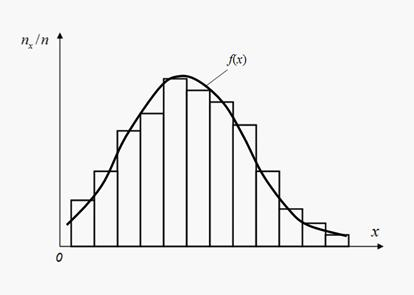Fig. 6. Histogram and theoretical density function

Thus random divergences connected with the limited volume of supervision, or divergences testifying about wrong selection of leveling function (hypothesis) will inevitably be found out. For the answer this question so-called «agreement criteria» are used. For this purpose random variable U describing a divergence of empirical and theoretical distributions in the assumption of the validity of theoretical distribution is entered. The measure of divergence U gets out so that function of its distribution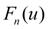did not depend on a kind of leveled (empirical) distribution and quickly enough converged on number of supervision n to limiting function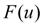. Then the actual degree of a divergence u is defined and the probability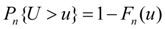is estimated. Small value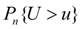speaks that the received divergence u by virtue of cleanly casual reasons is improbable, and theoretical distribution will badly be coordinated with empirical. However, high probabilities cannot be considered as the exhaustive proof of the validity of the theoretical law of distribution and testify only to absence of the bases it to reject.

Sometimes act differently: in advance count a measure of a divergence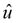which can be exceeded with the specified small probability, and at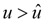the considered theoretical distribution is rejected.

There is a set of agreement criteria among which the most common are Pearson’s criterion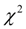and Kolmogorov-Smirnoff criterion .

In Pearson’s agreement criteriona measure of a divergence of theoretical and empirical distributions is the weighed sum of squares of deviations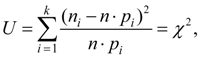(27)

where k – number of intervals of splitting of values of a random variable,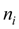– quantity of the observations which have got in the i -th interval,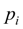– theoretical probability of occurrence of value from the i -th interval, n – general number of observations.

In practical problems it is recommended to have in each interval of splitting not less than 5-10 observations .

Let's designate through t number of the independent communications imposed on probability. Their general number is equal to quantity of characteristics of the theoretical distribution selected on empirical data, plus 1 (a condition of normalization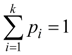). Thus, the scheme of application of criterionto an estimation of a coordination of theoretical and empirical distributions is reduced to the following:

1) Measure of a divergencefrom the formula (27) is defined.

2) Number of degrees of freedom r = k – t is defined.

3) On r andby means of special table  the probability of that value having distributionwith r by degrees of freedom, will surpass the given valueis defined.

If this probability is rather small, the hypothesis (a theoretical curve) is rejected as improbable. If this probability is rather great, it is possible to recognize a hypothesis not contradicting the received experimental data.

Be how much small owe probability р to reject or reconsider a hypothesis, is not solved on the basis of mathematical reasons and calculations. In practice if it appears, that р <0.1, it is recommended to check up or repeat experiment. If appreciable divergences will appear again, it is necessary to search for another law of distribution, more suitable for the description of empirical data. If the probability p > 0.1 (is rather great), it cannot be considered as the proof of validity of a hypothesis yet, and speaks only that the hypothesis does not contradict experimental data.

In Kolmogorov-Smirnoff criterion a measure of a divergence theoretical F ( x ) and empirical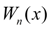distributions is the maximal module of a difference(28)

A.N.Kolmogorov has proved, that at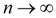irrespective of kind F ( x ) probability of an inequality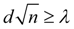(29)

aspires to the limit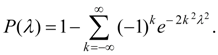(30)

For check of a hypothesis by Kolmogorov-Smirnoff criterion it is necessary to construct functions of distribution for theoretical F ( x ) and empiricaldistributions to define a maximum d of module of a difference between them and to find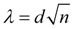. After this it is necessary to find probability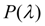from special table :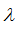0.0 1.000 0.7 0.711 1.4 0.040 0.1 1.000 0.8 0.544 1.5 0.022 0.2 1.000 0.9 0.393 1.6 0.012 0.3 1.000 1.0 0.270 1.7 0.006 0.4 0.997 1.1 0.178 1.8 0.003 0.5 0.964 1.2 0.112 1.9 0.002 0.6 0.864 1.3 0.068 2.0 0.001

The small probabilitytestifies to unacceptability of theoretical function.

 < Previous Contents Next >